# 第一次实验

/*A simple C program*/
#include <stdio.h>
int main(){
printf("202023332333\n");
return 0;
}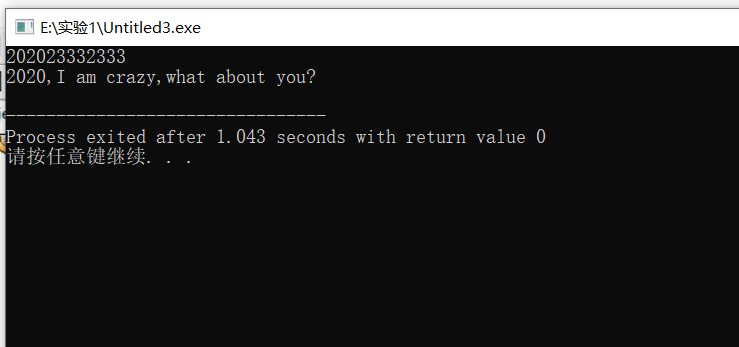/*循环打字符*/
#include <stdio.h>
int main (){
while(1)
printf("0 1");
return 0;
}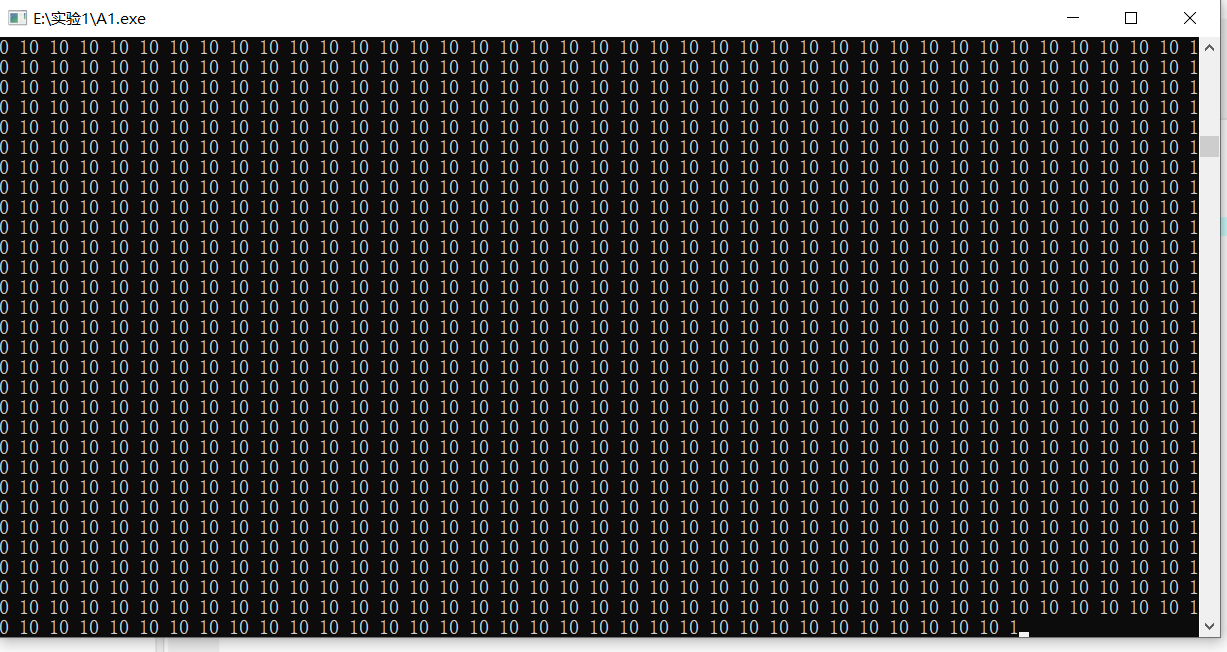/*循环打字符*/
#include <stdio.h>
int main (){
while(1)
printf("you are so beautiful");
return 0;
}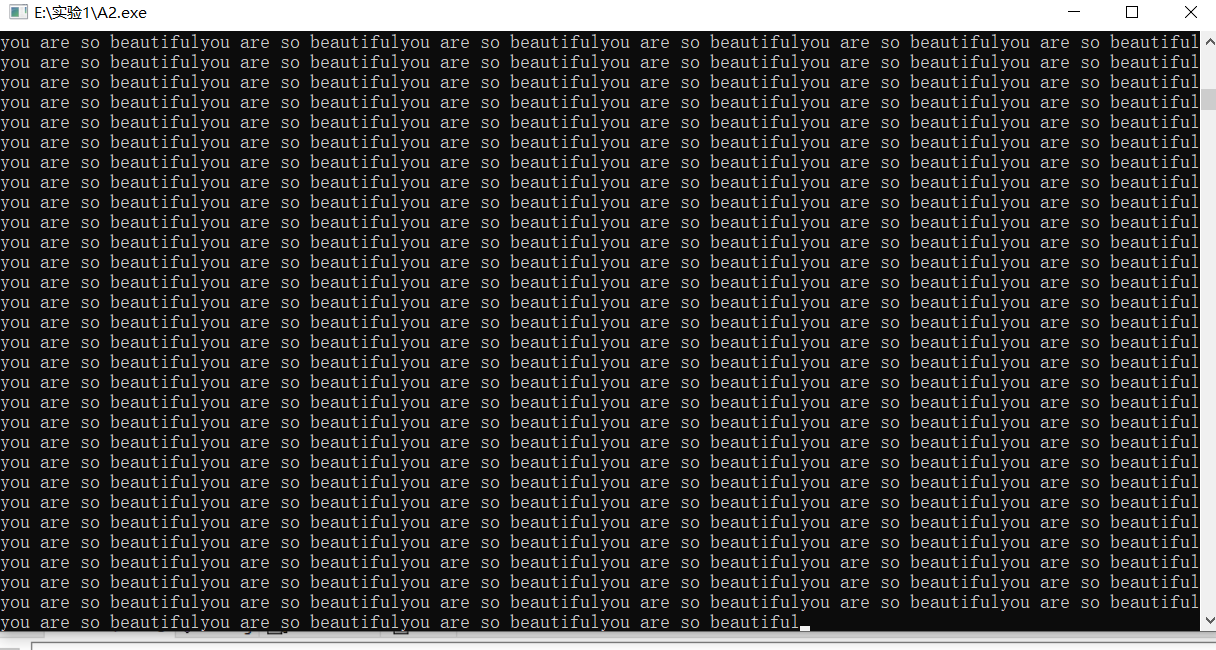/*用C语言实现一个简单的算术运算程序*/
#include <stdio.h>
int main(){
int x,y;
int r1,r2,r3,r4;
x=1;
y=2;
r1=x+y;
r2=x-y;
r3=x*y;
r4=x/y;

printf("r1=%d\n",r1);
printf("r2=&d\n",r2);
printf("r3=&d\n",r3);
printf("r4=%d\n",r4);
return 0;
}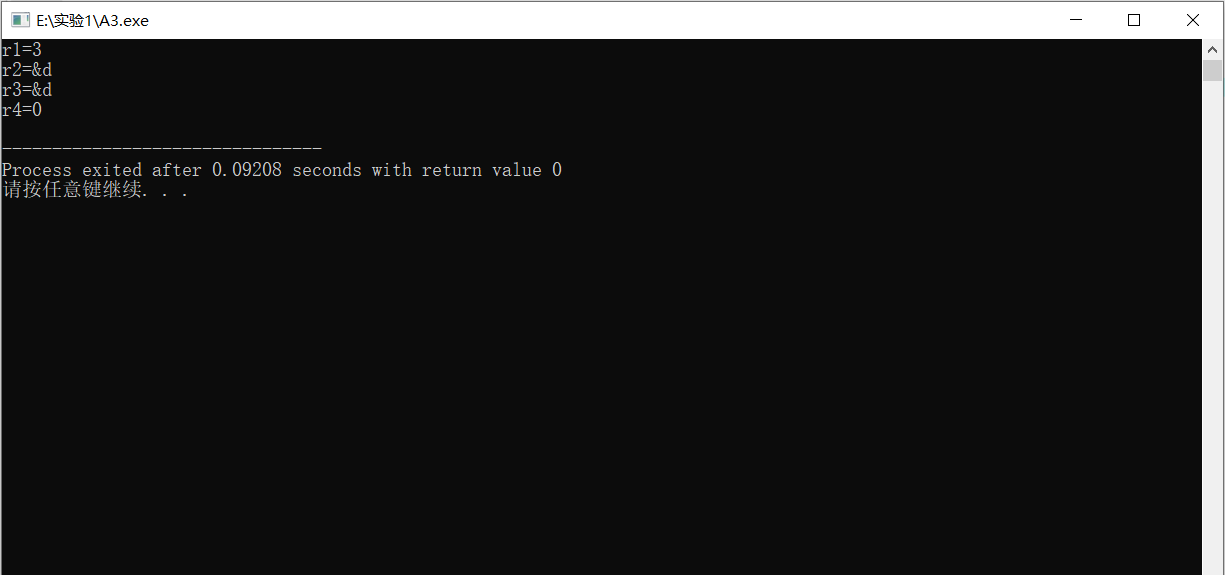/*用C语言实现一个简单的算术运算程序*/
#include <stdio.h>
int main(){
float x,y;
float r1,r2,r3,r4;
x=1;
y=2;
r1=x+y;
r2=x-y;
r3=x*y;
r4=x/y;

printf("r1=%f\n",r1);
printf("r2=&f\n",r2);
printf("r3=&f\n",r3);
printf("r4=%f\n",r4);
return 0;
}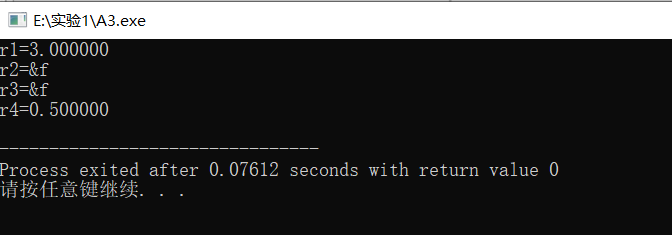//测试你是哪一类学习者
#include <stdio.h>
int main (){
char words;
printf("在家上学让一些人欢喜一些人忧.\n");
printf("1.作为一个宅，社恐，觉得这样很好。有网络，自主学习，很OK.\n");
printf("2.不喜，不喜，很不喜。眼睛快瞎了，脑壳有点卡...\n");
printf("3.中间派，不知道，说不清。\n");
printf("4.其它...\n");
printf("你的选择：");
printf("请补充说明你的想法：\n");
getchar();
gets(words);
}

printf("\n");
printf("当遇到问题时，你会选择：\n");
printf("1.遇到问题，就解决问题。不懂的，搜索式学习，逐个攻破.\n");
printf("2.遇到问题，不高兴。然后，。。。。，没有了.\n");
printf("3.其它。。。\n");
printf("你的选择；");

printf(":)\n");
printf("这样不能解决问题啊。。。我们选择1好不好：）\n");
printf("请补充说明你的想法：\n");
getchar();
gets(words);
}

return 0;
}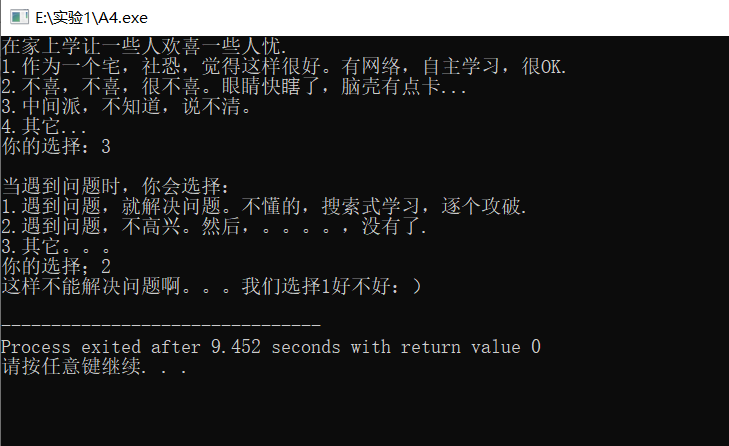posted @ 2020-10-17 15:50  I-AM-Y  阅读(18)  评论(0编辑  收藏